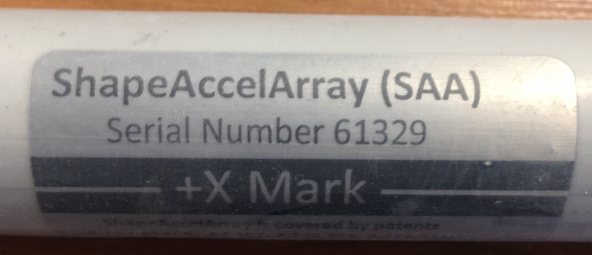For ShapeArrays (SAAs) without magnetometers that are used in vertical (3D) mode, in either ShapeArray™ Viewer (formerly known as SAAView) or SAACR_raw2data , the X-axis in the software is in the horizontal plane and by default has the same azimuth (heading) as the +X mark at the reference end of the ShapeArray (see Figure below).Figure 1: +X mark at the top end of a ShapeArray

In the general case the +X mark of the ShapeArray will be aligned at some known azimuth offset angle relative to a known direction. In these cases, it is convenient to use an azimuth offset angle in the software so that the X-axis represented in the software becomes the known direction. For details on setting azimuth offset angles for a ShapeArray, please refer to SAA X-Mark Protractor.

For vertical ShapeArray installations, the Y-axis is also in the horizontal plane and is rotated 90 degrees counter-clockwise from the X-axis, if you are looking down on the installation. The Z-axis is pointed vertically upward.

For horizontal (2D) installations, the naming of the axes differs depending on the software being used:

Horizontal Mode in SAARecorder:

1. The X-axis is aligned with the longitudinal dimension of the ShapeArray. For a perfectly horizontal ShapeArray of sensorized length L, the reference end is by default at X=0, and the non-reference end is at X=L.
2. The Z-axis is pointed up.
3. The Y-axis is perpendicular to both Z and X, but because the horizontal mode models the ShapeArray in a vertical plane, the Y-axis data will always be zero.

Horizontal Mode in SAAView:

1. The Z-axis is aligned with the longitudinal dimension of the ShapeArray. For a perfectly horizontal ShapeArray of sensorized length L, the reference end is by default at Z=0, and the non-reference end is at Z=L.
2. The X-axis is pointed up.
3. The Y-axis is perpendicular to both Z and X, but because the horizontal mode models the ShapeArray in a vertical plane, the Y-axis data will always be zero.

If the ShapeArray has one or more magnetometers and is being used in vertical (3D) mode, and no additional azimuth offset angle is specified, the X-axis in both ShapeArray™ Viewer and SAARecorder corresponds to magnetic north, and the Y-axis corresponds to magnetic west. The Z-axis points up.